## Curvature of a 1D curve in a 2D or 3D space

version 1.2.8 (85.5 KB) by
Calculates the local radius of curvature as well as the cumulative arc length and the curvature vector along a 1D curve in 2D or 3D space

Updated Wed, 10 Nov 2021 11:24:11 +0000

Function circumcenter finds the radius R and the center of the circumscribed circle of a triangle in 3D space.
Function curvature calls circumcenter for every triplet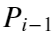,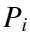,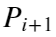of neighboring points along the curve. The curvature is defined as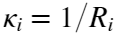. The curvature vector is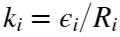, where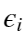is the unit vector in the direction fromto the center of the circle.
Note that this local calculation is sensitive to noise in the data.
The syntax is:
[L,R,K] = curvature(X)
X: array of column vectors for the curve coordinates. X may have two or three columns.
L: Arc length
K: Curvature vector

### Cite As

Are Mjaavatten (2022). Curvature of a 1D curve in a 2D or 3D space (https://www.mathworks.com/matlabcentral/fileexchange/69452-curvature-of-a-1d-curve-in-a-2d-or-3d-space), MATLAB Central File Exchange. Retrieved .

##### MATLAB Release Compatibility
Created with R2014b
Compatible with any release
##### Platform Compatibility
Windows macOS Linux